# How to use the DATE function in Excel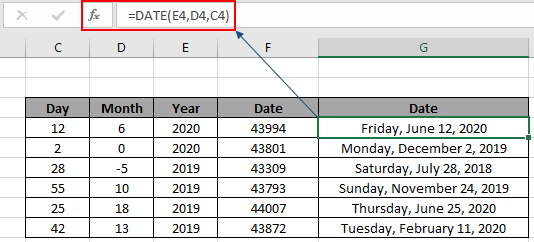The Excel DATE function is a DATE & TIME function. This function returns the DATE value in numerical format. Convert the output to date format via using Format cell option.

In this article, we will learn how to use the DATE function in Excel.

Syntax of DATE Function:

 =DATE(year,month,day)

year : valid numerical value for year

month : numerical value for month, can have zero and negative values

day : numerical value for day, can have zero and negative values

Example of DATE Function

All of these might be confusing to understand. So, let's test this formula via running it on the example shown below. So, here I have a table of some data. The first column contains the numeric values for the day value, second column contains month value and third contains the year value for the real date value as result.

Use the formula:

 =DATE(E4,D4,C4)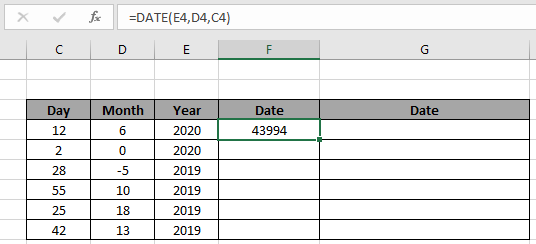It will return 43994. Here all the arguments to the DATE function are given using cell reference. As you can see the value is not in date format. So for that just change the format of the result cell to short or long date format as required.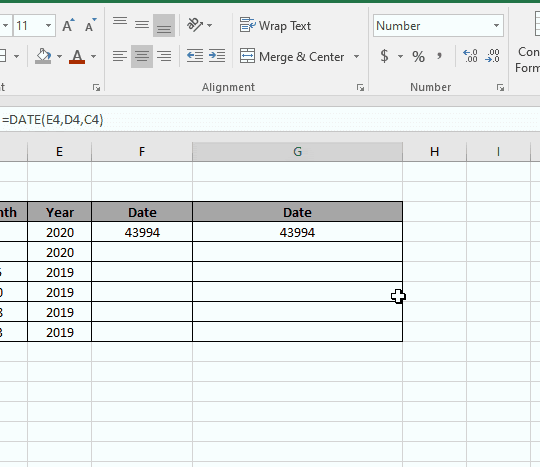As shown in the above gif that you can change the format of any cell to any format using the format cell option. Now You can copy the formula from one to the remaining cells using the Ctrl + D shortcut key or using the drag down option in excel.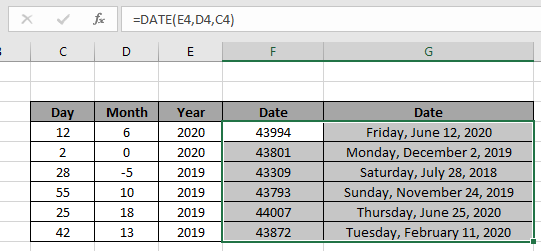As you can see from the above snapshot that DATE function takes day, month and year value as input and returns the whole date value. Negative or zero value as argument returns the date shifting respectively as per value.

Excel DATE function : DATE function with other formulas to return result in date format. DATE function is mostly used with other logic functions for calculating date values in Excel. From one date value extract month, day and year for more calculation like the one shown below to get the next anniversary date.

Use the formula:

 =DATE (YEAR (D5) + 1, MONTH(D5) , DAY(D5))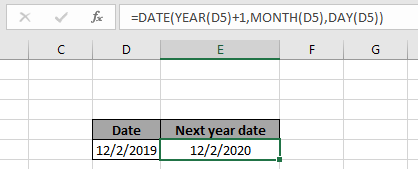Here the day function extract day , Month function extracts month and year function extracts year value from the date and perform operation over numerical values and return the arguments to the DATE function to return required date value.

Get nth day from date of year in Excel

Here we have some random date values and we need to find the nth day of year from the date value.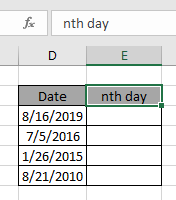Use the formula:

 = D3 - DATE ( YEAR ( D3 ) , 1 , 0 )

Explanation:

1. YEAR function returns the year value of the given date.
2. DATE function takes the argument and returns the last date of the previous year.
3. The subtraction operator takes the difference of the two and returns the elapsed date.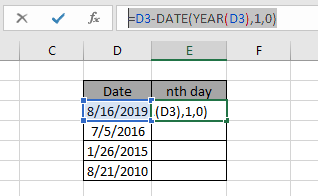Use the above function formulas to get the days between dates in Excel.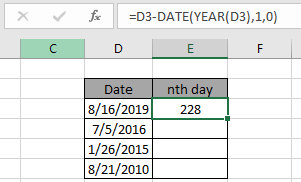As you can see the formula works fine as it returns 228 as result. Now use drag drop down till the dataset dates.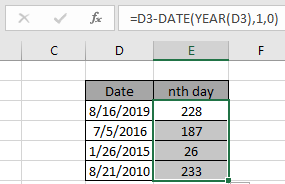Here are the days from the date of the year calculated using the formula.

We can calculate the same result using one more logic. If we subtract the given date value from the first date of the same year and add 1 ( +1 ).

Generic formula:

 = date - DATE ( YEAR ( date ) , 1 , 1 ) + 1

You can use any of the formulas as both return the same result.

Note: DO NOT give the date argument directly to the formula as Excel formula doesn't necessarily understand the date value and returns error. Provide the date argument using the DATE function or using the cell reference option.

EDATE function example

Here we have a list of dates of birth with Emp ID.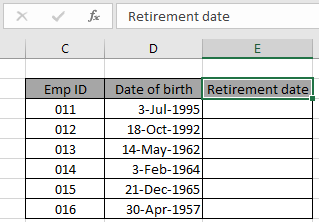Here we need to find the retirement date from the given DAte of birth values.

Use the formula in E3 cell:

 = EDATE ( B3 , 12*60 )Here the date value is given as cell reference. Press Enter to get the retirement date.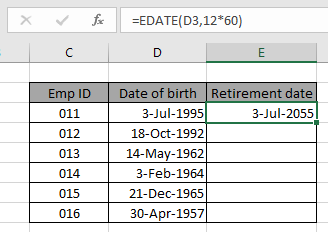As you can see the retirement date for the 011 Emp ID comes out to be July 3rd, 2055. Now copy the formula in other cells using the drag down option or using the shortcut key Ctrl + D as shown below.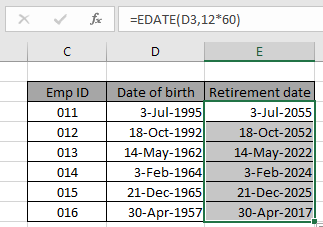As you can see we obtained all employees' retirement age using the Excel EDATE function. Generally the returned date value is not in the required date format. Use the format cell option or Ctrl + 1 as shown in the below snapshot.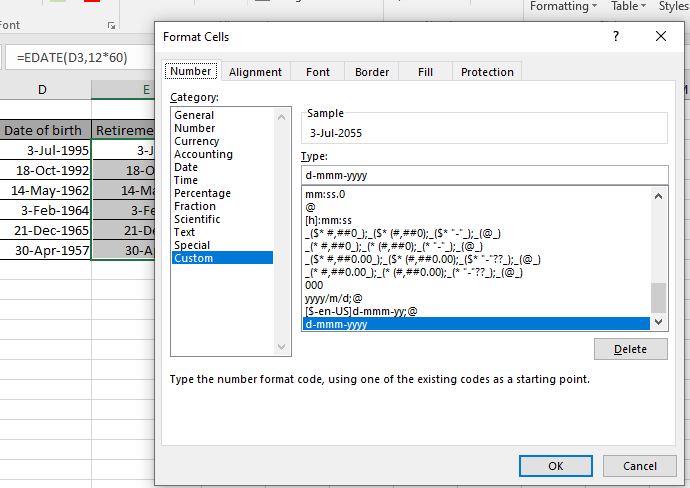Here are some observational notes shown below.

Notes:

1. The formula only works with numbers.
2. Arguments to the function must be numeric values or else the function returns #NAME? Error.
3. No argument to the function returns #NUM! Error.
4. Only No year argument returns the year value as 1900.
5. MONTH and DAY arguments can be zero or negative. As day argument 1 returns 1st date of month, 0 input will return the just before date value from the previous month.

Hope this article about How to use the DATE function in Excel is explanatory. Find more articles on DATE & TIME formulas here. If you liked our blogs, share it with your fristarts on Facebook. And also you can follow us on Twitter and Facebook. We would love to hear from you, do let us know how we can improve, complement or innovate our work and make it better for you. Write to us at info@exceltip.com.

Related Article

How to calculate age from date of birth | returns the year age as numerical value from the date value comparing it with today's date value.

SUM if date is between | Returns the SUM of values between given dates or period in excel.

Sum if date is greater than given date | Returns the SUM of values after the given date or period in excel.

2 Ways to Sum by Month in Excel | Returns the SUM of values within a given specific month in excel.

Popular Articles:

50 Excel Shortcuts to Increase Your Productivity | Get faster at your task. These 50 shortcuts will make you work even faster on Excel.

How to use the VLOOKUP Function in Excel | This is one of the most used and popular functions of excel that is used to lookup value from different ranges and sheets.

How to use the COUNTIF function in Excel 2016 | Count values with conditions using this amazing function. You don't need to filter your data to count specific values. Countif function is essential to prepare your dashboard.

How to Use SUMIF Function in Excel | This is another dashboard essential function. This helps you sum up values on specific conditions.

Terms and Conditions of use

The applications/code on this site are distributed as is and without warranties or liability. In no event shall the owner of the copyrights, or the authors of the applications/code be liable for any loss of profit, any problems or any damage resulting from the use or evaluation of the applications/code.